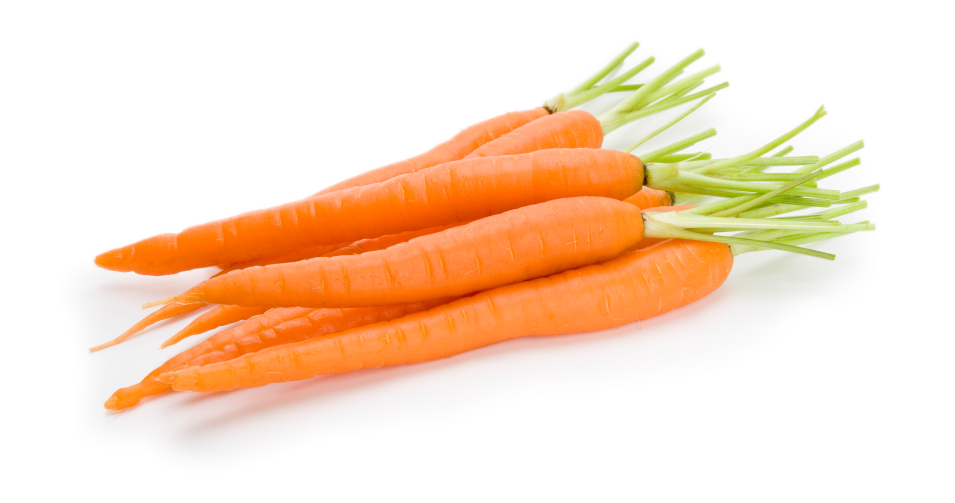# I am carrot +_+

Probability Level 4We have a magical carrot multiplier machine, which multiplies an input carrot into at most 6 carrots, and minimum just 1 (gives the carrot back). But it can multiply only original carrots, the ones which were not put into the machine ever.

There are $286$ carrots of $143$ different shades such that there are exactly $2$ carrots of each shade. All of these carrots after treating with the machine, are filled in a bag.

There are $11^n$ different compositions possible for this bag. Find $n$.

Details and Assumptions:-

$\bullet$ The carrots formed by multiplying one particular carrot are identical (due to magic of the multiplier).

$\bullet$ The $143$ different shades are well distinguishable, no 2 look alike.

This problem is a part of the set Vegetable Combinatorics

×